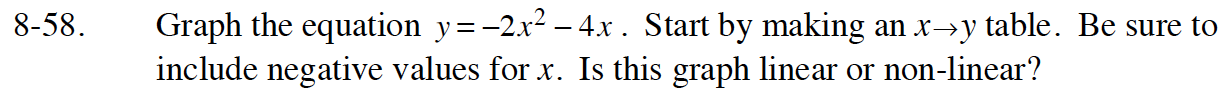Home > ACC7 > Chapter cc38 > Lesson cc38.2.1 > Problem8-58

8-58.

Graph the equation y = −2x2 − 4x. Start by making an x → y table. Be sure to include negative values for x. Is this graph linear or non-linear? 8-58 HW eTool (Desmos). Homework Help ✎Remember that linear means there is only one value of y for each value of x .

Complete the table in the eTool below to graph the equation.
Click the link at right for the full version of the eTool: CC3 8-58 HW eTool